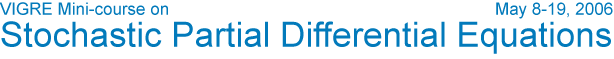Robert C. Dalang's Abstract Speakers Schedule & Notes Participants Visitor Information Contact Home Title: The stochastic wave equation Abstract. The stochastic wave equation in spatial dimensions greater than 1 presents interesting challenges that are not present in the study of the stochastic heat equation. For instance, the kernel does not have a strong regularizing property and its irregularity increases with the dimension.We shall discuss issues of existence of solutions for linear and non-linear forms of the equation with various kinds of driving noises, with an emphasis on spatially homogeneous Gaussian noise. For spatial dimensions 2 and 3, we will discuss Hölder-Sobolev regularity estimates. If time allows, we will also discuss some potential-theoretic results. Prerequisites. Some familiarity with Walsh's martingale measure stochastic integral, as presented in [W; Chapter 2], and, some familiarity with a basic s.p.d.e. in spatial dimension 1 (heat or wave equation), as can be found in [W; Chapter 3]. [W] Walsh, J. B. An introduction to stochastic partial differential equations, Ecole d'été de Probabilités de Saint Flour XIV, Lecture Notes in Mathematics, Vol. 1180, Springer Verlag, 1986. VIGRE       U of U Math